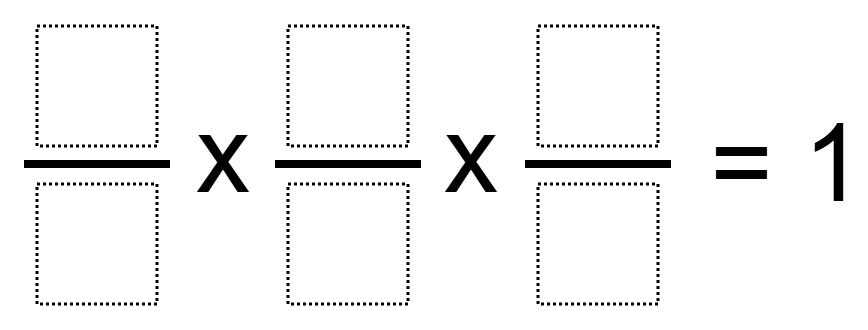# Multiplying 3 Fractions to Get 1

Directions: Using the digits 1 to 9, at most one time each, place a digit in each box to make a true statement.### Hint

Could some of the fractions have a value greater than 1?

3/4 * 6/9 * 2/1 = 1

Source: Owen Kaplinsky, Answer by Joseph Nguyen

## Add Fractions with Decimal Sums

Directions: Using the digits 1 to 9 at most one time each, place a digit …

1.4/1 x 1/2 x 1/2

2.4/1 x 1/2 x 1/2 = 1

3.4/1 x 1/2 x 1/2=1

4.i got 3/4 x 6/9 x 2/1 = 1

5.3/4 x 6/9 x 2/1 = 1

6.One of my students did: 6/9 x 4/8 x 3/1

7.Excellent for factors and multiples lessons.

8.9.One of my students found 1/6×9/3×8/4=1

10.3/4 * 6/5 * 10/9

11.9/3 * 1/6 * 4/2 = 1

9/3 * 1/6 8 8/4 = 1